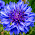6. Explain about image sampling and quantization process.

6. Explain about image sampling and quantization process.

Image Sampling and Quantization:

The output of most sensors is a continuous voltage waveform whose amplitude and spatial
behavior are related to the physical phenomenon being sensed. To create a digital image, we
need to convert the continuous sensed data into digital form. This involves two processes:
sampling and quantization.

Basic Concepts in Sampling and Quantization:

The basic idea behind sampling and quantization is illustrated in Fig.6.1. Figure 6.1(a) shows a
continuous image, f(x, y), that we want to convert to digital form. An image may be continuous
with respect to the x- and y-coordinates, and also in amplitude. To convert it to digital form, we
have to sample the function in both coordinates and in amplitude. Digitizing the coordinate
values is called sampling. Digitizing the amplitude values is called quantization.

The one-dimensional function shown in Fig.6.1 (b) is a plot of amplitude (gray level) values of
the continuous image along the line segment AB in Fig. 6.1(a).The random variations are due to
image noise. To sample this function, we take equally spaced samples along line AB, as shown
in Fig.6.1 (c).The location of each sample is given by a vertical tick mark in the bottom part of
the figure. The samples are shown as small white squares superimposed on the function. The set
of these discrete locations gives the sampled function. However, the values of the samples still
span (vertically) a continuous range of gray-level values. In order to form a digital function, the
gray-level values also must be converted (quantized) into discrete quantities. The right side of
Fig. 6.1 (c) shows the gray-level scale divided into eight discrete levels, ranging from black to
white. The vertical tick marks indicate the specific value assigned to each of the eight gray
levels. The continuous gray levels are quantized simply by assigning one of the eight discrete
gray levels to each sample. The assignment is made depending on the vertical proximity of a
sample to a vertical tick mark. The digital samples resulting from both sampling and quantization
are shown in Fig.6.1 (d). Starting at the top of the image and carrying out this procedure line by
line produces a two-dimensional digital image.

Sampling in the manner just described assumes that we have a continuous image in both
coordinate directions as well as in amplitude. In practice, the method of sampling is determined
by the sensor arrangement used to generate the image. When an image is generated by a single sensing element combined with mechanical motion, as in Fig. 2.13, the output of the sensor is
quantized in the manner described above. However, sampling is accomplished by selecting the
number of individual mechanical increments at which we activate the sensor to collect data.
Mechanical motion can be made very exact so, in principle; there is almost no limit as to how
fine we can sample an image. However, practical limits are established by imperfections in the
optics used to focus on the sensor an illumination spot that is inconsistent with the fine resolution achievable with mechanical displacements. When a sensing strip is used for image acquisition, the number of sensors in the strip establishes the sampling limitations in one image direction. Mechanical
motion in the other direction can be controlled more accurately, but it makes little sense to try to
achieve sampling density in one direction that exceeds the sampling limits established by the
number of sensors in the other. Quantization of the sensor outputs completes the process of
generating a digital image.

Fig.6.1. Generating a digital image (a) Continuous image (b) A scan line from A to Bin the
continuous image, used to illustrate the concepts of sampling and quantization (c) Sampling
and quantization. (d) Digital scan line

When a sensing array is used for image acquisition, there is no motion and the number of sensors
in the array establishes the limits of sampling in both directions. Figure 6.2 illustrates this
concept. Figure 6.2 (a) shows a continuous image projected onto the plane of an array sensor.
Figure 6.2 (b) shows the image after sampling and quantization. Clearly, the quality of a digital
image is determined to a large degree by the number of samples and discrete gray levels used in
sampling and quantization.

Fig.6.2. (a) Continuos image projected onto a sensor array (b) Result of image
sampling and quantization.

1.2.3.